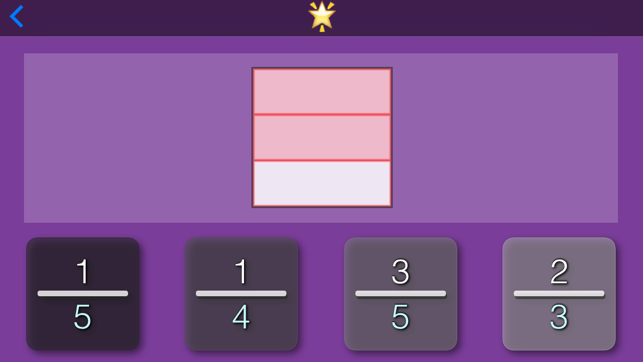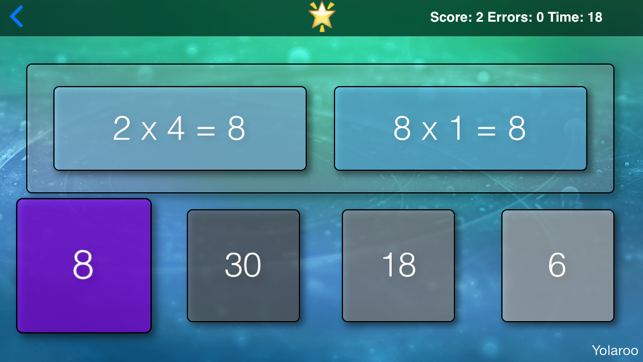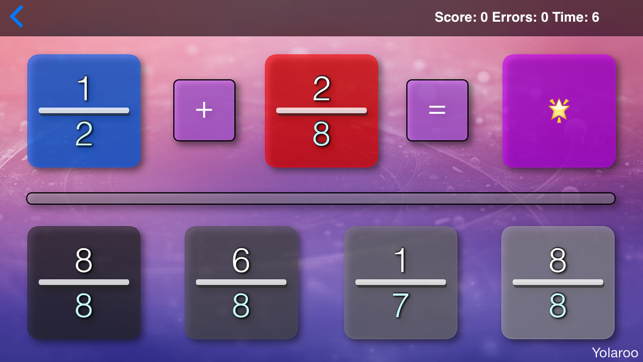## Screenshots

•••## Description

Yolaroo Fractions is a great teaching tool to help teach or learn both fractions (a/b) and least common multiples LCM(a,b)

Fractions are difficult for students to learn, because there are many steps and rules that must be followed to properly solve a fraction.

This app has six activities that make learning fractions easy and simple.

- This app has an an introduction to equivalent multiples. This makes learning least common multiples easier.

- This app has a LCM Challenge mode that helps the student learn least common multiples. After answering many problems quickly and easily the brain naturally learns how to apply the equation:

lcm (a,b) = |a*b| / gcd(a,b).

After having learned how to easily calculate least common multiples the student can then easily see how to properly solve fractions by properly using least common multiples as needed to make common denominators.

- This app has a 'Fractionator' that shows the equivalence relationship between fractions. This app lets students see the answer for a large number of fraction problems while the brain of the student learns the patterns of mathematics quickly and naturally. With this tool the student can easily see the different rules used for the addition, the subtraction, the multiplication, and the division of fractions. This makes learning math as easy as learning a native language and not as if the student is having to learn something esoteric that remains to be solved.

- This app has a basic fraction game that has students learn the relation between graphical fractions and the numerical
fractions.

- This app has a fractional equivalence game that helps students learn to recognize equivalent fractions.

- This app has a Fraction Challenge mode that tests the student's ability to answer simple and advanced fractions. This helps the student learn how to solve fractions with an infinite workbook and also acts as a test to show that the student can solve fractions.

Everyone needs a good education. This app is 100% free, because learning and education are important for everyone.

## What’s New

Version 2.0

Three new activities:
- Simple multipliers
- Graphical fractions
- Equal fraction recognition

iOS 7 update
Graphic update

4.7 out of 5
3 Ratings

3 Ratings

## Information

Seller
Matthew Moscatello
Size
20.7 MB
Category
Education
Compatibility

Requires iOS 7.0 or later. Compatible with iPhone, iPad, and iPod touch.

Languages

English

Age Rating
4+
Price
Free

## Supports

•